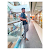New update is available. Click here to update.
Last Updated: Jun 30, 2023
Easy

# How do you get the logical XOR of two variables in Python0 upvotes

## Introduction

Hello there,

Over here, you will be learning about the bitwise operator xor of two variables using Python. We all know what logical operators are, the AND, OR, NOT, and XOR. If you are unaware of what operators are, you can refer to this article, Operators. This article will discuss one of the Bitwise operators in Python, i.e., the Python XOR operator.

“ Recommended Topic, Python Round Function, Swapcase in Python”.

## Python XOR operator

The XOR operator is abbreviated as the exclusive OR operator. This operator compares two bitwise binary numbers.In this operator, when both the input values are the same, the output value is 0. When both the input values are different, the output value is 1.

But when two integer values are the operator's input, the integers are converted into binary values. The operator is applied, and the resultant value is converted back into an integer.

You can also check out the other Bitwise operatorsFibonacci Series in Python

### Bitwise Python XOR operator Table

Here is a table to portray how the Python XOR operator works for binary values.

#### Example of Python XOR operator of Two Variables

``````a = 14 = 1110(Binary)
b = 6 = 0110(Binary)
a ^ b = 1110^0110
= 1000
= 8 (Decimal)``````

#### Program in  Python

``````a = 14
b = 6
#Printing bitwise Python XOR operator
print("a ^ b =", a ^ b)``````

Output:

``a ^ b = 8``

In this program above:

The value of a 14 is converted into a binary number "1110," and 6 is converted into the binary number "0110". The bitwise operators are applied here to get the value "1000," giving the decimal value 8.

You can compile it with online python compiler.

Now, let's discuss some FAQs related to Python XOR operators.

### What are operators?

Operators are specific symbols that provide logical and computational functions in the program.

### What are the types of operators?

There are 7 types of operators: arithmetic operators, assignment operators, comparison operators, logical operators, identity operators, membership operators, and bitwise operators.

### Why are operators used in Python?

Operators are used for applying a logical sense in the programs. It is usually taken in binary values or true/false values.

### Are operators present in all programming languages?

Yes, since it is the one that provides logical computation sense to the programs, it is not only present; it is essential while programming.

## Conclusion

We have discussed the Python XOR operator with some examples and some FAQs related to the Python XOR operator.

After reading about the Python XOR operator, are you not feeling excited to read/explore more articles on Data Structures and Algorithms? Don't worry; Coding Ninjas has you covered. See GraphBinary TreeBFS vs DFSCheck for Balanced Parentheses in an Expression, and Stack to learn.

Check out this problem - XOR Queries On Tree